##### Six Sigma For DummiesFrom a quality perspective, Six Sigma is defined as 3.4 defects per million opportunities. This figure is called a Six Sigma level of quality. Sigma scores are thrown about so much that you definitely need to be comfortable understanding what they are and how they’re calculated. Basically, a sigma score tells you how many standard deviations can fit between the mean and specification limit of any process or specification.

The sigma score can be applied to the performance of anything that has a specification and a defect rate: the performance of the mail system in delivering letters to the correct address, the ability of an automobile manufacturer to produce a door that fits to the body within a required dimensional tolerance, or a repeated budgeting process that must be completed within its specified schedule window.

## How many standard deviations can fit?

The central tendency of the performance distribution is defined by its mean. The amount of variation in the performance, or the width of the distribution, is defined by its standard deviation σ. The question is how many standard deviations can you fit between the process or characteristic’s mean and its specification limit SL?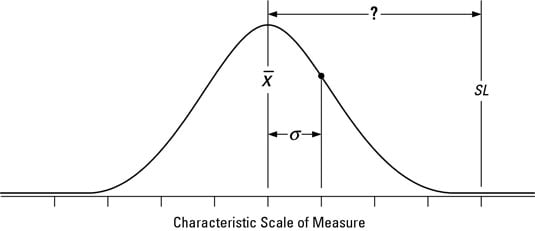You can see that four standard deviations can fit between the mean and the specification limit. The exact number can always be calculated by the formula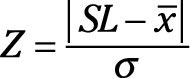A low sigma (Z) score means that a significant part of the tail of the distribution extends past the specification limit. So the higher the sigma (Z) score, the fewer the defects. A process or characteristic gets a good sigma (Z) score when the variation distribution is safely away from the edge of the specification cliff. A sigma (Z) score can change in one of three ways:

• The location of the central tendency of the distribution — the mean — moves either closer or farther from the specification limit.

• The width of the distribution, as defined by the standard deviation σ changes.

• The location of the specification limit SL moves either closer or farther from the characteristic or process variation.

## Compare short-term to long-term sigma score calculations

From the mean and the standard deviation, you can calculate a sigma (Z) score. A wrinkle here is that you must know what type of standard deviation you’re using to calculate the sigma (Z) score: Is it a short-term standard deviation σST, or is it a long-term standard deviation σLT?

If you’re using a short-term standard deviation, the sigma (Z) score you calculate is a short-term sigma score ZST: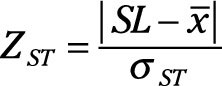If, however, you have a long-term standard deviation, you can calculate the long-term sigma score ZLT: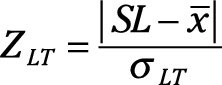## Link short-term capability to long-term performance with the 1.5-sigma shift

Short-term variation performance, as quantified by the short-term sigma score ZST, represents the best variation performance that you can expect out of your currently configured process. It’s an idealistic measure of capability. It’s also the easiest type of data to collect; just go and quickly grab a relatively small sample of measurements from the process or characteristic, and you have it.

But in the long term, a process or characteristic doesn’t operate ideally like it does in the short term. Its performance will degrade by shift, drift, and trend influences. At the heart of Six Sigma is a method that combines the best of both worlds. It allows you to leverage the economy of short-term variation data while projecting realistic, long-term performance versus the process’s or characteristic’s specifications.

The characteristic or process stays within specifications during the short term and appears to have no problems. But over the long term, disturbances to the process cause it to expand, and this expansion creates defects beyond the specification limit.

One mathematical way to simulate the effect of these degrading, long-term influences is to artificially move the short-term distribution closer to the specification limit until the amount of defects for the short-term distribution is the same as that for the long-term distribution.

Early practitioners of Six Sigma proposed that mathematically shifting a characteristic’s or process’s short-term distribution closer to its specification limit by a distance of 1.5 times its short-term standard deviation would approximate the number of defects occurring in the long term. This breakthrough idea can be applied directly to the calculation of short-term and long-term sigma (Z) scores.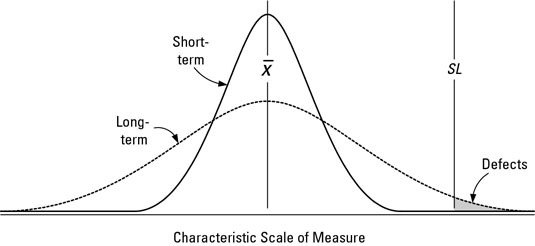Because ZST represents the number of short-term standard deviations between the variation center and the specification, the sigma (Z) score of the shifted distribution is

Zshifted = ZST – 1.5

But with the shifted distribution being equivalent, defect-wise, to the long-term distribution, the preceding equation can be rewritten as

ZLT = ZST – 1.5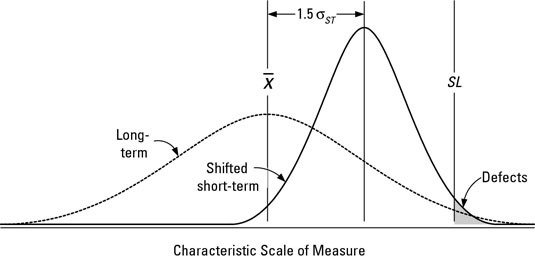So what Six Sigma practitioners do is measure the short-term variability of a process or characteristic and calculate its short-term sigma score. Then they immediately translate this score to the expected long-term defect rate performance, using the 1.5 short-term standard deviation shift. This long-term sigma score, ZLT, is communicated in terms of defects per million opportunities, DPMO.